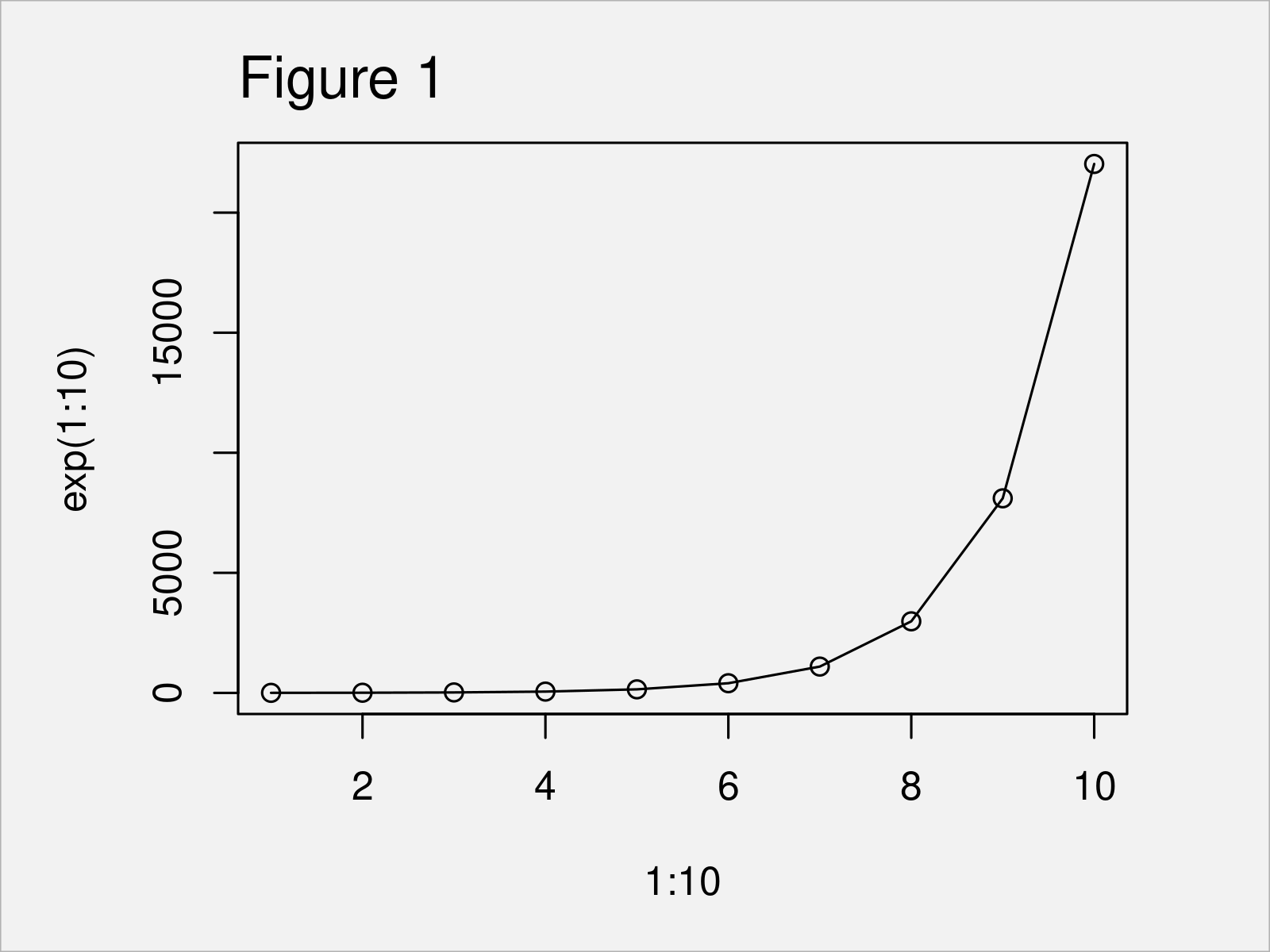# exp Function in R (4 Examples)

This tutorial shows how to compute exponentials using the exp function in R.

The content looks as follows:

## Example 1: Basic Application of the exp() Function

This example demonstrates how to compute the exponential of a numeric value using the exp function in R.

Let’s assume that we want to calculate the exponential of the value 5. Then, we can apply the exp function as shown below:

```exp(5)                              # Apply exp function
#  148.4132```

The previous output of the RStudio console shows the result: The exponential of 5 is 148.4132.

## Example 2: Apply exp() Function to Vector Object

In this example, I’ll illustrate how to use the exp command to calculate the exponential of all elements in a vector object.

Have a look at the following R syntax and its output:

```exp(1:10)                           # Apply exp function to vector
#       2.718282     7.389056    20.085537    54.598150   148.413159
#     403.428793  1096.633158  2980.957987  8103.083928 22026.465795```

The result of the previous code is a vector containing ten values. Each of these values corresponds to one of the values in our input vector.

## Example 3: Draw Output of exp() Function in Point & Line Plot

The following code shows how to visualize the exponential function in a graphic.

For this, we can use the plot, lines, and exp functions as illustrated below:

```plot(x = 1:10,                      # Plot output of exp function
y = exp(1:10))
lines(x = 1:10,                     # Add lines to plot
y = exp(1:10))```As shown in Figure 1, the previous R syntax has drawn a point and line chart of the exponential values of our input vector.

## Example 4: Basic Application of the expm1() Function

The R programming language provides another function for the computation of exponential values minus 1, which is called expm1.

This function calculates exp(x) – 1 accurately, even for |x| ＜＜ 1.

In this example, I’ll show how to apply the expm1 to a vector containing a range from 1 to 10 (i.e. the same vector as in Example 2):

```expm1(1:10)                         # Apply expm1 function
#       1.718282     6.389056    19.085537    53.598150   147.413159
#     402.428793  1095.633158  2979.957987  8102.083928 22025.465795```

The output is the same as the output in Example 2 minus the value 1´.

## Video, Further Resources & Summary

If you need more explanations on the topics of this article, I recommend having a look at the following video on my YouTube channel. In the video tutorial, I demonstrate the R programming syntax of this tutorial.

Furthermore, you could read the related tutorials on this homepage. You can find some tutorials below:

In this R programming tutorial you have learned how to apply the exp function. In case you have additional questions, tell me about it in the comments section.

Subscribe to the Statistics Globe Newsletter